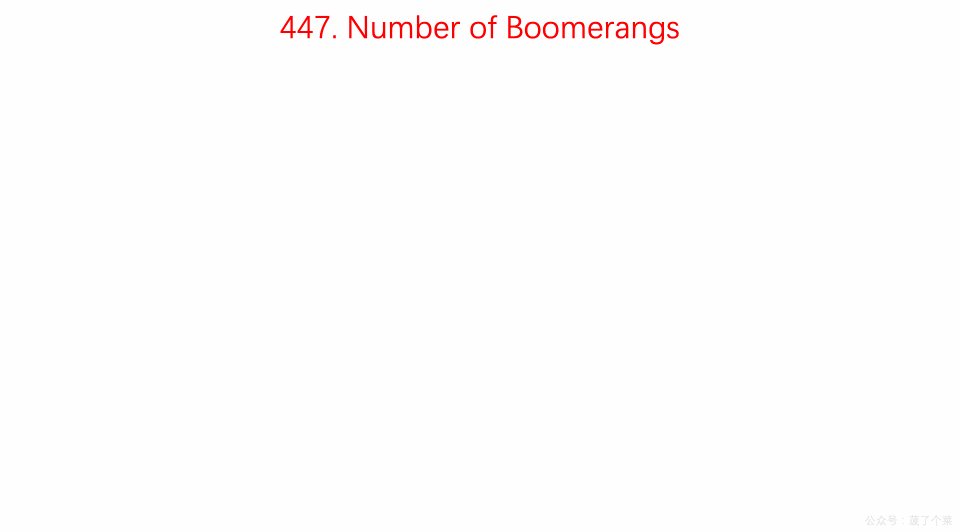# LeetCode 第 447 号问题：回旋镖的数量

### 题目描述

``````输入:
[[0,0],[1,0],[2,0]]

2

``````

### 题目解析

n 最大为 500，可以使用时间复杂度为 O(n^2)的算法。

• 遍历所有的点，让每个点作为一个锚点
• 然后再遍历其他的点，统计和锚点距离相等的点有多少个
• 然后分别带入 n(n-1) 计算结果并累加到res中
##### Tips：
###### Tip1
• 如果有一个点 a，还有两个点 b 和 c ，如果 ab 和 ac 之间的距离相等，那么就有两种排列方法 abc 和 acb；
• 如果有三个点 b，c，d都分别和a之间的距离相等，那么有六种排列方法，abc, acb, acd, adc, abd, adb；
• 如果有 n 个点和点 a 距离相等，那么排列方式为 n(n-1)。
###### Tip2
• 计算距离时不进行开根运算, 以保证精度；
• 只有当n大于等于2时，res值才会真正增加，因为当n=1时，增加量为`1*(1-1)=0`

### 动画描述### 代码实现

``````// 447. Number of Boomerangs
// https://leetcode.com/problems/number-of-boomerangs/description/
// 时间复杂度: O(n^2)
// 空间复杂度: O(n)
class Solution {
public:
int numberOfBoomerangs(vector<pair<int, int>>& points) {

int res = 0;
for( int i = 0 ; i < points.size() ; i ++ ){

// record中存储 点i 到所有其他点的距离出现的频次
unordered_map<int, int> record;
for(int j = 0 ; j < points.size() ; j ++){
if(j != i){
// 计算距离时不进行开根运算, 以保证精度
record[dis(points[i], points[j])] += 1;
}
}

for(unordered_map<int, int>::iterator iter = record.begin() ; iter != record.end() ; iter ++){
res += (iter->second) * (iter->second - 1);
}
}
return res;
}

private:
int dis(const pair<int,int> &pa, const pair<int,int> &pb){
return (pa.first - pb.first) * (pa.first - pb.first) +
(pa.second - pb.second) * (pa.second - pb.second);
}
};

``````